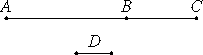# Proposition 28

If two numbers are relatively prime, then their sum is also prime to each of them; and, if the sum of two numbers is relatively prime to either of them, then the original numbers are also relatively prime.

Let two relatively prime numbers AB and BC be added.

I say that their sum AC is also relatively prime to each of the numbers AB and BC.

If CA and AB are not relatively prime, then some number D measures CA and AB.VII.Def.12

Since then D measures CA and AB, therefore it also measures the remainder BC. But it also measures BA, therefore D measures AB and BC which are relatively prime, which is impossible.

Therefore no number measures the numbers CA and AB. Therefore CA and AB are relatively prime. For the same reason AC and CB are also relatively prime. Therefore CA is relatively prime to each of the numbers AB and BC.

Next, let CA and AB be relatively prime.

I say that AB and BC are also relatively prime.

If AB and BC are not relatively prime, then some number D measures AB and BC.

VII.Def.12

Now, since D measures each of the numbers AB and BC, therefore it also measures the whole CA. But it measures AB, therefore D measures CA and AB which are relatively prime, which is impossible.

Therefore no number measures the numbers AB and BC. Therefore AB and BC are relatively prime.

Therefore, if two numbers are relatively prime, then their sum is also prime to each of them; and, if the sum of two numbers is relatively prime to either of them, then the original numbers are also relatively prime.

Q.E.D.

## Guide

This proposition is used in IX.15.